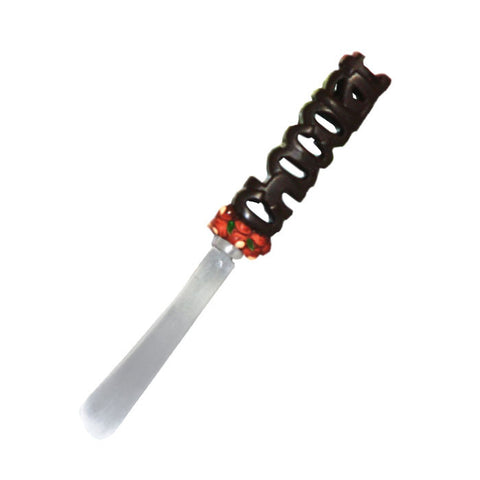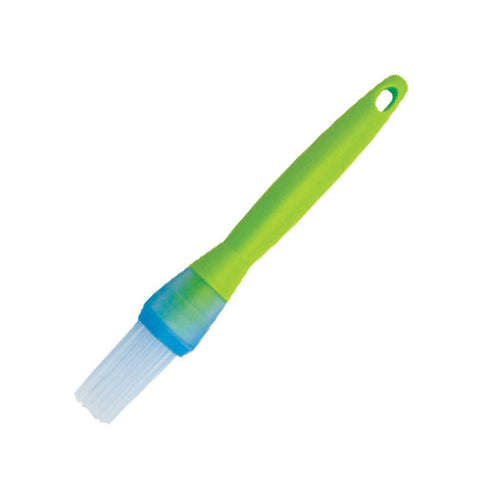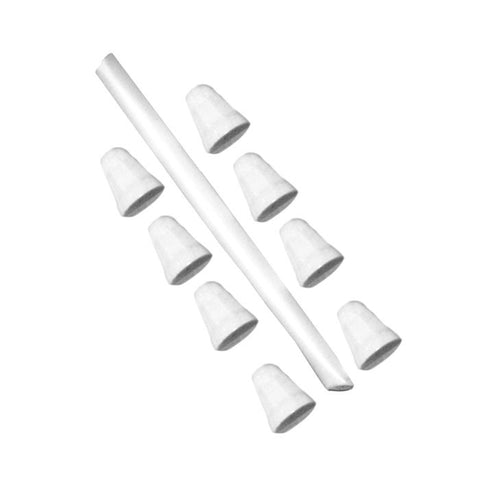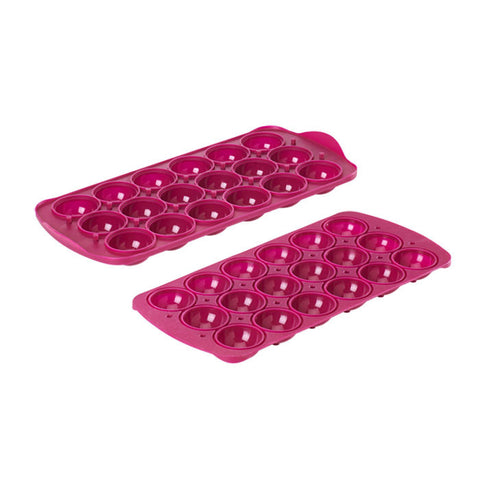# Measuring Cups

• 6 pieces
• ABS, Hi Temp
• Colour Coded
• Includes: Scraper

Measurements on Scraper:

 FLUID VOLUME MEASUREMENT DRY VOLUME 1 fluid oz. (2 tbsp.) =30mL 1/2 tsp. =2.5mL 4 fluid oz. (1/2 cup) =120mL 1tsp. = 5mL 8 fluid oz. (1cup) =240mL 1 tbsp. =15mL 12 fluid oz. (1 1/2 cup) =360mL 1 cup =240mL 16 fluid oz. (2cups) =480mL 4 cup =1qt.=946mL

Pin It

## Related Items